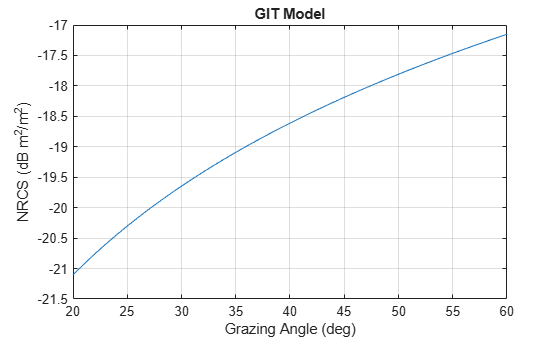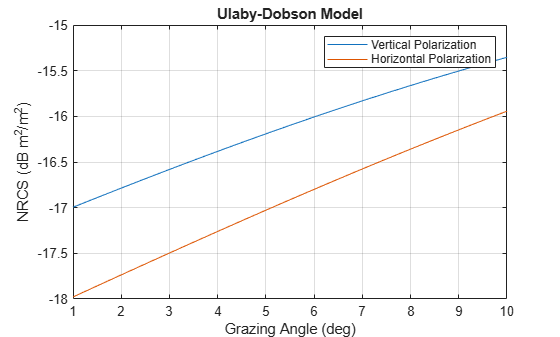# surfaceReflectivityLand

Normalized reflectivity of land surface

## Description

Normalized reflectivity is the radar cross-section of a unit area of a land surface. Multiplying by the total area of a surface or the illuminated area of a surface gives the total radar cross-section. Normalized reflectivity is also referred to as surface σ0 and is a function of frequency and grazing angle.

To compute the normalized reflectivity:

1. Create the `surfaceReflectivityLand` object and set its properties.

2. Call the object with arguments, as if it were a function.

## Creation

### Syntax

``refl = surfaceReflectivityLand``
``refl = surfaceReflectivityLand(Name=Value)``

### Description

example

````refl = surfaceReflectivityLand` creates a normalized reflectivity object `refl` for a land surface. Use this object to generate a normalized radar cross section (NRCS). This syntax creates a normalized reflectivity object with a `'Barton'` land `Model` and a `'Flatland'` `LandType`.```

example

````refl = surfaceReflectivityLand(Name=Value)` creates a normalized reflectivity object for a land surface with the specified property `Name` set to the specified `Value`. You can specify additional name-value pair arguments in any order as (`Name1=Value1`, … ,`NameN=ValueN`).Example: ```refl = surfaceReflectivityLand(Model="GIT",LandType="Soil",SurfaceHeightStandardDeviation=1)``` creates a normalized reflectivity object for land using the GIT model with a `LandType` of `Soil` and a `SurfaceHeightStandardDeviation` of 1.```

## Properties

expand all

Unless otherwise indicated, properties are nontunable, which means you cannot change their values after calling the object. Objects lock when you call them, and the `release` function unlocks them.

If a property is tunable, you can change its value at any time.

Land reflectivity model, specified as `'Barton'`, `'APL'`, `'Billingsley'`, `'GIT'`, `'Morchin'`, `'Nathanson'`, `'Nathanson'`, `'UlabyDobson'`, or `'ConstantGamma'`. Descriptions of the models and land types are shown in the table Land Reflectivity Models and Land Types.

Land type, specified as a char or string. The allowable land type and their default values depend on the `Model` property. If the `Model` property is not specified, the default land type is `'Flatland'`. Descriptions of the models and land types are shown in the table Land Reflectivity Models and Land Types.

Data Types: `char` | `string`

Standard deviation of the surface height, specified as a positive scalar. Units are in meters.

#### Dependencies

To enable this property, set the `Model` property to `'GIT'`.

Data Types: `double`

Polarization of reflectivity model, specified as `'H'` or `'V'`. `'H'` designates horizontal polarization and `'V'` designates vertical polarization.

#### Dependencies

To enable this property, set the `Model` property to `'UlabyDobson'`.

Data Types: `char` | `string`

Terrain gamma value used in the constant gamma clutter reflectivity model, specified as a scalar. The gamma value depends on both terrain type and the operating frequency. The default value is representative of flat land. Units are in dB.

Example: -15

#### Dependencies

To enable this property, set the `Model` property to `'ConstantGamma'`.

Data Types: `double`

Speckle distribution type, specified as `'None'`, `'Lognormal'`, `'Rayleigh'`, `'Weibull'`, or `'Custom'`. Speckle is a multiplicative factor used to make clutter data appear noisier and is especially applicable to imaging applications.

Speckle is correlated with clutter RCS and is applied as I = σ*n, where σ represents the clutter RCS and n represents random numbers, which are often drawn from an independent identically-distributed unity mean noise statistical distribution.

• `None` – No speckle is applied.

• `Lognormal` – Speckle has a lognormal distribution. Define the distribution using the `SpeckleMean` and `SpeckleStandardDeviation` properties. Default values of these properties create speckle with a normalized mean lognormal distribution.

• `Rayleigh` – Speckle has a Rayleigh distribution. Define the distribution using the `SpeckleScale` property. The default value of this property creates speckle with a unit mean Rayleigh distribution.

• `Weibull` – Speckle has a Weibull distribution. Define the distribution using the `SpeckleScale` and `SpeckleShape` properties. The default values of these properties create speckle with a unit mean Rayleigh distribution.

Data Types: `char` | `string`

Mean value of lognormal-distributed speckle, specified as a scalar.

#### Dependencies

To enable this property, set the `Speckle` property to `'Lognormal'`.

Data Types: `double`

Standard deviation of lognormal-distributed speckle, specified as a non-negative scalar.

#### Dependencies

To enable this property, set the `Speckle` property to `'Lognormal'`.

Data Types: `double`

Scale parameter for speckle for the Rayleigh and Weibull distributions, specified as a positive scalar.

#### Dependencies

To enable this property, set the `Speckle` property to `'Rayleigh'` or `'Weibull'`.

Data Types: `double`

Shape value for the Weibull speckle distribution, specified as a positive scalar.

#### Dependencies

To enable this property, set the `Speckle` property to `Weibull`.

Data Types: `double`

## Usage

### Syntax

``nrcs = refl(graz,freq)``

### Description

example

````nrcs = refl(graz,freq)` returns the normalized radar cross section `nrcs` at grazing angle `graz` and frequency `freq`. When the `Model` property is set to `'Billingsley'`, `graz` is interpreted as a depression angles.```

### Input Arguments

expand all

Grazing or depression angle of a surface relative to the radar, specified as a scalar or an M-length row vector of real values. When the land `Model` property is set to `'Billingsley'`, the angle is interpreted as a depression angle `depressionang` between –90° and 90°. For all other models, the angle is interpreted as a grazing angle `grazingang` ranging from 0° to 90° . Units are in degrees.

Transmitted frequencies, specified as a positive scalar or N-length vector of positive values. Units are in Hz.

Example: `freq = 7*10e9`

### Output Arguments

expand all

Normalized surface reflectivity, returned as either a real-valued N-length row vector or a real-valued M-by-N matrix. Normalized reflectivity is also called normalized radar cross section. M is the length of the grazing angle or depression angle vector `graz` and N is the length of the frequency vector `freq`. `nrcs` is dimensionless but often expressed as m²/m².

## Object Functions

To use an object function, specify the System object™ as the first input argument. For example, to release system resources of a System object named `obj`, use this syntax:

`release(obj)`

expand all

 `step` Run System object algorithm `release` Release resources and allow changes to System object property values and input characteristics `reset` Reset internal states of System object

## Examples

collapse all

Plot the normalized radar cross-section for grazing angles from 5 to 90 degrees. Assume the default 'Barton' land `Model` and 'Flatland' `LandType`. Set the radar frequency to 1 GHz.

```grazAng = 5:90; freq = 1e9; reflectivity = surfaceReflectivityLand; nrcs = reflectivity(grazAng,freq); plot(grazAng,pow2db(nrcs)) grid on xlabel('Grazing Angle (deg)') ylabel('NRCS (dB m^2/m^2)') title('Barton Land Model with Flat Land Type')```Configure a `radarscenario` to simulate a reflective land surface. Add a land surface object to define the physical properties of the scenario surface. The surface is a simple 200-by-200 meter rectangle. Use the `surfaceReflectivityLand` function to create a constant-gamma reflectivity model with a gamma value of -10 dB. Use the scenario `landSurface` method to add the rectangular land region and the radar reflectivity model to the scenario. Use a surface reference height of 16 meters.

```scene = radarScenario(UpdateRate = 0, IsEarthCentered = false); refl = surfaceReflectivityLand(Model = "ConstantGamma", Gamma = -10); srf = landSurface(scene,RadarReflectivity = refl, ... Boundary=[-100 100; -100 100],ReferenceHeight = 16)```
```srf = LandSurface with properties: RadarReflectivity: [1×1 surfaceReflectivityLand] ReflectivityMap: 1 ReferenceHeight: 16 Boundary: [2×2 double] Terrain: [] ```

Create a normalized reflectivity object using the GIT 'Model' and a 'Soil' land type. Obtain the normalized radar cross-section at a frequency of 3 GHz over grazing angles from 20 to 60 degrees. Assume a surface height standard deviation of two meters. Plot the surface reflectivity.

```grazAng = 20:60; freq = 10e9; reflectivity = surfaceReflectivityLand(Model="GIT", ... LandType="Soil",SurfaceHeightStandardDeviation=2); nrcs = reflectivity(grazAng,freq); plot(grazAng,pow2db(nrcs)) grid on xlabel('Grazing Angle (deg)') ylabel('NRCS (dB m^2/m^2)') title('GIT Model')```Create a normalized reflectivity object using the Billingsley 'Model' and a 'LowReliefRural' land type. Obtain the normalized radar cross-section at a frequency of 3 GHz over depression angles from 0.1 to 3 degrees. Plot the surface reflectivity.

``` depAng = 0.1:0.1:2; freq = 3e9; reflectivity = surfaceReflectivityLand(Model="Billingsley", ... LandType="LowReliefRural"); nrcs = reflectivity(depAng,freq); plot(depAng,pow2db(nrcs)) grid on xlabel('Depression Angle (deg)') ylabel('NRCS (dB m^2/m^2)') title('Billingsley Model')```Create a normalized reflectivity object using the Ulaby-Dobson model for a grass land type. Obtain the normalized radar cross-section for both vertical and horizontal polarizations at a frequency of 10 GHz over grazing angles from 1 to 10 degrees. Plot the surface reflectivities.

```grazAng = 1:0.1:10; freq = 10e9; reflectivity_v = surfaceReflectivityLand(Model="UlabyDobson", ... LandType="Grass",Polarization="V"); nrcs_v = reflectivity_v(grazAng,freq); reflectivity_h = surfaceReflectivityLand(Model="UlabyDobson", ... LandType="Grass",Polarization="H"); nrcs_h = reflectivity_h(grazAng,freq); plot(grazAng,pow2db(nrcs_v)) hold on plot(grazAng,pow2db(nrcs_h)) grid on legend('Vertical Polarization','Horizonal Polarization') xlabel('Grazing Angle (deg)') ylabel('NRCS (dB m^2/m^2)') title('Ulaby-Dobson Model')```Create a surface with two hills. Plot the surface on a 200-by-200 meter grid with grid points one meter apart. Add the surface to a radar scenario. Assume the surface has a radar reflectivity defined by a constant gamma model.

```[x,y] = meshgrid(linspace(-100,100,201)); ht1 = 40*exp(-(x.^2 + y.^2)/30^2); ht2 = 100*exp(-((x-60).^2 + y.^2)/25^2); ht = ht1 + ht2; p = surfc(x(1,:),y(:,1),ht); axis equal axis tight shading interp simTime = 3; scene = radarScenario(UpdateRate = 1, ... IsEarthCentered = false,StopTime = simTime); gammaDB = surfacegamma('Flatland'); refl = surfaceReflectivityLand(Model = 'ConstantGamma',Gamma = gammaDB); srf = landSurface(scene,RadarReflectivity = refl, ... Terrain = ht,Boundary = [-100,100;-100,100]);```

Use surface manager to identify the surface.

`scene.SurfaceManager`
```ans = SurfaceManager with properties: UseOcclusion: 1 Surfaces: [1×1 radar.scenario.LandSurface] ```
`scene.SurfaceManager.Surfaces`
```ans = LandSurface with properties: RadarReflectivity: [1×1 surfaceReflectivityLand] ReflectivityMap: 1 ReferenceHeight: 0 Boundary: [2×2 double] Terrain: [201×201 double] ```

Obtain and plot the height of the surface at the point (50,-30).

```xt = 50; yt = -30; htx = height(srf,[xt,yt])```
```htx = 21.1046 ```
```hold on plot3(xt,yt,htx+5,'ow','MarkerFaceColor','r') xlabel('x') ylabel('y') hold off```expand all

 Barton, David Knox. Radar Equations for Modern Radar. Artech House, 2013.

 Long, Maurice W. Radar Reflectivity of Land and Sea. 3rd ed, Artech House, 2001.

 Nathanson, Fred E., et al. Radar Design Principles: Signal Processing and the Environment. 2. ed., Repr, Scitech Publ, 2004.

 Reilly, J. P., R. L. McDonald, and G. D. Dockery. "RF-Environment Models for the ADSAM Program." Report No. A1A97U-070, Laurel, MD: Johns Hopkins University Applied Physics Laboratory, August 22, 1997.

 Billingsley, J. Barrie. Low-Angle Radar Land Clutter: Measurements and Empirical Models. William Andrew Pub. : SciTech Pub. ; Institution of Electrical Engineers, 2002.

 Richards, M. A., et al., editors. Principles of Modern Radar. SciTech Pub, 2010.

 Morchin, Fred E., J. Patrick Reilly, and Marvin Cohen. Radar Design Principles: Signal Processing and the Environment. 2nd ed. New York: McGraw-Hill, 1991.

 Ulaby, Fawwaz T., and M. Craig Dobson. Handbook of Radar Scattering Statistics for Terrain. Artech House, 1989.